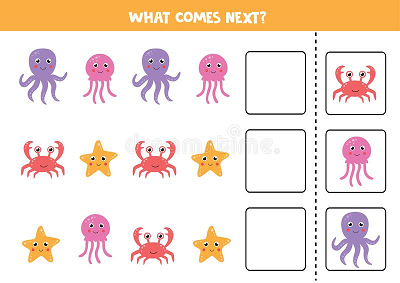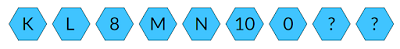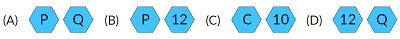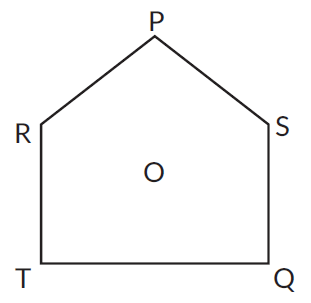Math & Beyond

# What is Logical Sequence and Ranking?

6.2k views

 1 Introduction 2 What is Logical Sequence? 3 Practical examples 4 Examples to try 5 Learning outcomes 6 Conclusion 7 Frequently Asked Questions (FAQs) 8 External References

29 December 2020

What is ranking?

What is logical sequence?

Logical sequence means, a sequence of instructions, that have been designed to be executed one after the other.

It forms the part of reasoning ability. As the name suggests, we need to think logically.## What is Logical Sequence and Ranking

A thread identifies a single control flow, which is a logical sequence of instructions, within a process. By logical sequence of instructions, we mean a sequence of instructions that have been designed to be executed one after the other one.

What is Logical Sequence and Ranking? is mentioned below in the Downloadable PDF.

## What is Logical Sequence?

A logical sequence is a set of numbers, words, objects etc, following in a sequence with some sort of relation between two consecutive sets.  Sometimes, it is also called  a progression.

The logical conclusion or result of a series of facts or events is the only one which can come from the given pattern, according to the rules of logic. Something that is logical seems reasonable or sensible in the circumstances.

This definition leads us to sequences like an arithmetic sequence, wherein, the consecutive numbers have a constant difference, a geometric sequence wherein, the consecutive numbers have a constant ratio,and so on.

Logical reasoning (verbal reasoning) refers to the ability of a student to understand and logically work through concepts and problems expressed in words. It checks the ability of a student to work and implement the exact words that are asked in the question. The logic is expressed verbally, and you have to understand the logic before solving the questions.

Practice online quizzes fully solved examples with detailed answers. Logical sequence of words is all about Consistent arrangement which is the important course of action of words.

• Alpha-numeric sequence
• Number Sequence
• Ranking Test
• Time sequence test
• Logical sequence of words
• Alphabet Test examples.

### Best practices to follow:

• Observe the alphabets given in each question.
• Try to find the pattern or sequence it follows.
•  Decipher the code assigned to each alphabet.
•  Figure out the rule followed by the given arrangement of alphabets/words.
•  Always refer to the given options to get a sense of the answer to be found.

## Practical examples of Logical Sequence and Ranking

Example: Find the missing value(B) P 12

Example: How many odd numbers are there in the given series which are immediately preceded and immediately followed by an even number?

5 1 4 7 3 9 8 5 7 2 6 3 1 5 8 6 3 8 5 2 2 4 3 4 9 6

(A) 1              (B) 2         (C) 3         (D) 4

 (D) 4

Example: P, Q, R, S and T are five friends sitting facing point O as shown in the figure. If P and T interchange their positions and then Q and R interchange their positions, then which of the following is true?A) R is to the left of P.                                   (B) Q is second to the right of R.
(C) T is second to the left of P.                     (D) S is to the right of T.

 (C) T is second to the left of P.

Example: How many possible and meaningful words can be made with the second, the sixth, the ninth and the twelfth letters of the word "CONTRIBUTION"? If more than one such word can be made, give M as the answer and if no such word can be formed, give X as the answer and if there is only one word then give O as the answer.

(A) N             (B) O             (C) M             (D) X

 (B) 0

Example: Arrange the following word in a meaningful order.
1. Karnataka
2. Universe
3. Bengaluru
4.World
5. India

(A) 3, 1, 4, 5, 2       (B) 1, 3, 5, 4, 2        (C) 3, 1, 5, 4, 2       (D) 3, 1, 2, 4, 5

 (C) 3, 1, 5, 4, 2

Example: If every third letter from the following English alphabet is dropped, which letter will be seventh to the right of the eleventh letter from the right?

A B C D E F G H I J K L M N O P Q R S T U V W X Y Z

(A) V           (B) U           (C) K            (D) I

 (A) V

## Try out these examples!

Question 1: If 1st November is Sunday, then which day will be 1st December?

(A) Monday         (B) Tuesday        (C) Wednesday        (D) Thursday

Question 2: Satish remembers that his brother's birthday is after the 15th but before
the 18th of February. His sister Kajal remembers that her brother's birthday is after the 16th but before the 19th of February. On which day is Satish's brother's birthday?

(A) 16th February        (B) 17th February        (C) 18th February        (D) 19th February

Question 3: Arrange the given words in the sequence in which they occur in the dictionary and then choose the correct sequence.
1. Eagle 2. Earth 3. Eager 4. Early 5. Each

(A) 1, 5, 2, 4, 3        (B) 2, 1, 4, 3, 5        (C) 2, 3, 5, 4, 1         (D) 5, 3, 1, 4, 2

Question 4: Answer the below question based on the information given below.
Six friends are sitting around a circular table at equal distances from each other. Sita sits two places right of Gita who is exactly opposite to Nita. Nita is on the immediate left of Lata, who is exactly opposite to Rita. Mita is sitting between Lata and Gita.
Which of the following is NOT TRUE about Sita's position?

A) Between Rita and Nita

(B) Opposite to Mita

(C) Left of Rita

(D) All of the above

Question 5: How many 8's are there in the given series, which are immediately preceded by a number which does not divide it but followed by a number which divides it?

4 8 2 8 2 8 3 8 5 8 8 5 3 2 8 2 3 8 4 7 1 5 8 3 8 2 8 6 8 8 6

(A) 1        (B) 2        (C) 3        (D) 4

## Learning outcomes

• Figure out the pattern used in alphabets and numbers.
• Arranging the given data in sequential order and simplifying it.
• Using certain simple tricks to solve the question according to the given options.

## Conclusion

Logical reasoning checks the ability of a student to work and implement the exact words that are asked in the question. The logic is expressed verbally, and you have to understand the logic before solving the questions.

Practice online quizzes fully solved examples with detailed answers. Logical sequence of words is all about Consistent arrangement which is the important course of action of words.

Cuemath, a student-friendly mathematics and coding platform, conducts regular Online Live Classes for academics and skill-development, and their Mental Math App, on both iOS and Android, is a one-stop solution for kids to develop multiple skills. Understand the Cuemath Fee structure and sign up for a free trial.

## 1. What is a logical sequence?

Logical sequence of words as the name implies is that type of reasoning which consists of words and we have to find out a sequence which is logical in that context.

## 2. What is order and ranking?

Ranking and order is an important topic under logical reasoning section that involves an arrangement of position or ranks of an object or a person either from left to right or top to bottom.

## External References

Related Articles
Award-winning math curriculum, FREE for a year